# Background subtraction – OpenCV

Background subtraction is a way of eliminating the background from image. To achieve this we extract the moving foreground from the static background.

Background Subtraction has several use cases in everyday life, It is being used for object segmentation, security enhancement, pedestrian tracking, counting the number of visitors, number of vehicles in traffic etc. It is able to learn and identify the foreground mask.

In OpenCV we have 3 algorithms to do this operation –

BackgroundSubtractorMOG – It is a Gaussian Mixture-based Background/Foreground Segmentation Algorithm.

BackgroundSubtractorMOG2 – It is also a Gaussian Mixture-based Background/Foreground Segmentation Algorithm. It provides better adaptability to varying scenes due illumination changes etc.

BackgroundSubtractorGMG – This algorithm combines statistical background image estimation and per-pixel Bayesian segmentation.

How to apply OpenCV in-built functions for background subtraction –
Step #1 – Create an object to signify the algorithm we are using for background subtraction.
Step #2 – Apply `backgroundsubtractor.apply()` function on image.

Below is the Python implementation for Background subtraction –

 `# importing libraries ` `import` `numpy as np ` `import` `cv2 ` ` `  `# creating object ` `fgbg1 ``=` `cv2.bgsegm.createBackgroundSubtractorMOG();    ` `fgbg2 ``=` `cv2.createBackgroundSubtractorMOG2(); ` `fgbg3 ``=` `cv2.bgsegm.createBackgroundSubtractorGMG(); ` ` `  `# capture frames from a camera  ` `cap ``=` `cv2.VideoCapture(``0``); ` `while``(``1``): ` `    ``# read frames ` `    ``ret, img ``=` `cap.read(); ` `     `  `    ``# apply mask for background subtraction ` `    ``fgmask1 ``=` `fgbg1.``apply``(img); ` `    ``fgmask2 ``=` `fgbg2.``apply``(img); ` `    ``fgmask3 ``=` `fgbg3.``apply``(img); ` `     `  `    ``cv2.imshow(``'Original'``, img); ` `    ``cv2.imshow(``'MOG'``, fgmask1); ` `    ``cv2.imshow(``'MOG2'``, fgmask2); ` `    ``cv2.imshow(``'GMG'``, fgmask3); ` `    ``k ``=` `cv2.waitKey(``30``) & ``0xff``; ` `    ``if` `k ``=``=` `27``: ` `        ``break``; ` ` `  `cap.release(); ` `cv2.destroyAllWindows(); `

Output: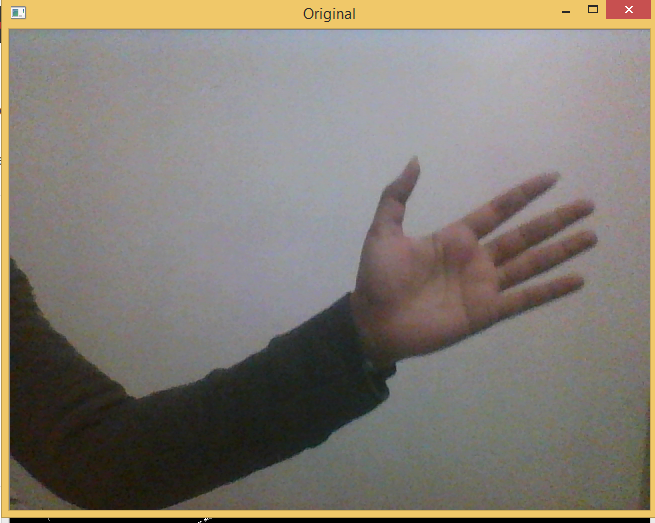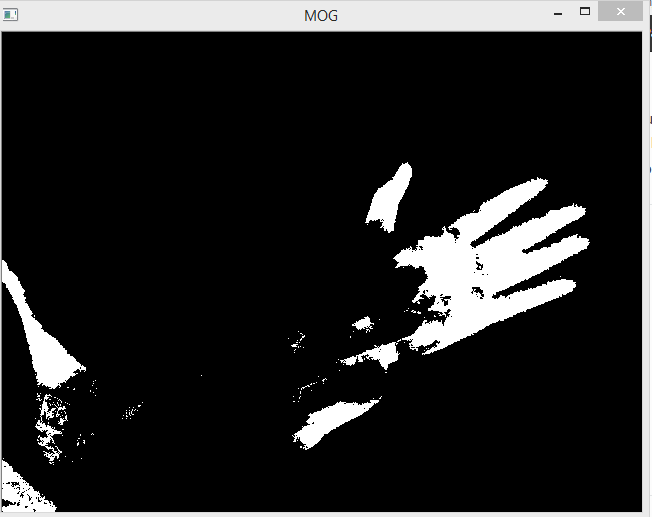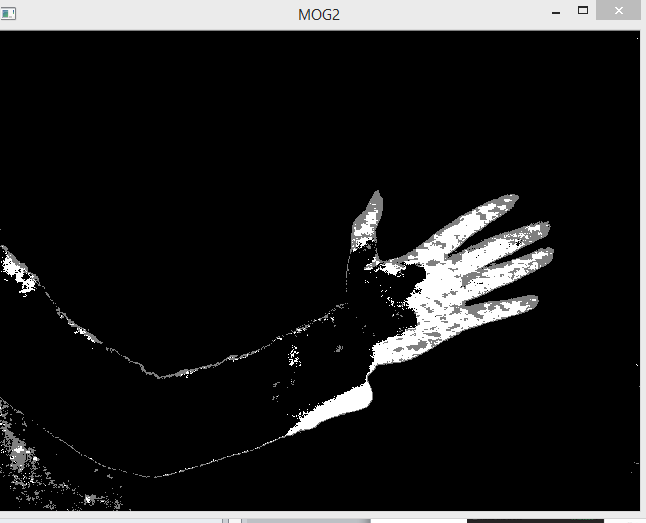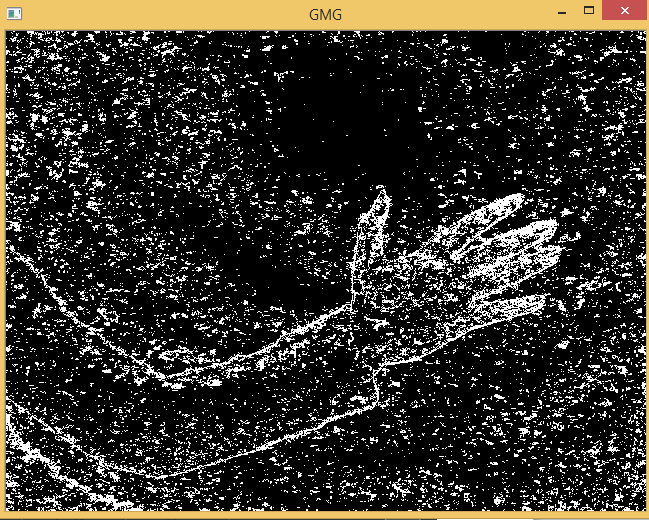We can see that there is a lot of noise in the resultant image for `BackgroundSubtractorGMG`, hence it is always preferred to use morphological transformation to the result to remove the noises.

 `# importing libraries ` `import` `numpy as np ` `import` `cv2 ` ` `  `kernel ``=` `cv2.getStructuringElement(cv2.MORPH_ELLIPSE, (``3``, ``3``)); ` ` `  `# creating object ` `fgbg ``=` `cv2.bgsegm.createBackgroundSubtractorGMG(); ` ` `  `# capture frames from a camera  ` `cap ``=` `cv2.VideoCapture(``0``); ` `while``(``1``): ` `    ``# read frames ` `    ``ret, img ``=` `cap.read(); ` `     `  `    ``# apply mask for background subtraction ` `    ``fgmask ``=` `fgbg.``apply``(img); ` `     `  `    ``# with noise frame ` `    ``cv2.imshow(``'GMG noise'``, fgmask); ` `     `  `    ``# apply transformation to remove noise ` `    ``fgmask ``=` `cv2.morphologyEx(fgmask, cv2.MORPH_OPEN, kernel); ` `     `  `    ``# after removing noise ` `    ``cv2.imshow(``'GMG'``, fgmask); ` `     `  `    ``k ``=` `cv2.waitKey(``30``) & ``0xff``; ` `    ``if` `k ``=``=` `27``: ` `        ``break``; ` ` `  `cap.release(); ` `cv2.destroyAllWindows(); `

Output: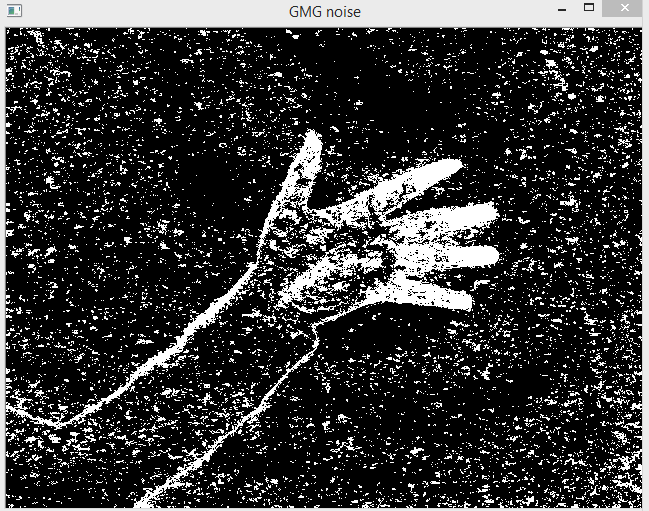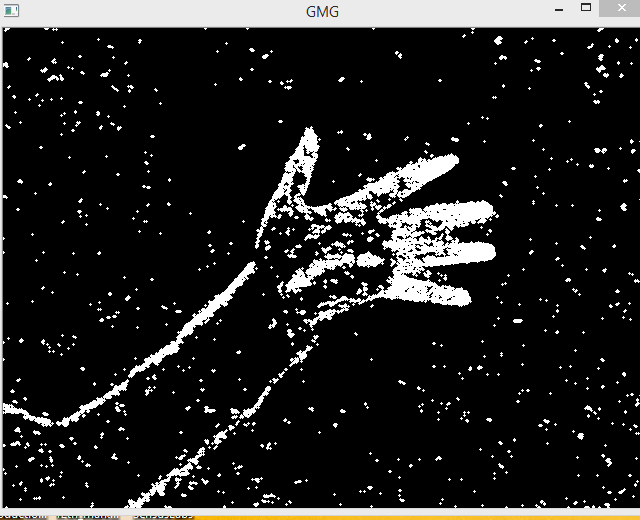Attention geek! Strengthen your foundations with the Python Programming Foundation Course and learn the basics.

To begin with, your interview preparations Enhance your Data Structures concepts with the Python DS Course.

My Personal Notes arrow_drop_upCheck out this Author's contributed articles.

If you like GeeksforGeeks and would like to contribute, you can also write an article using contribute.geeksforgeeks.org or mail your article to contribute@geeksforgeeks.org. See your article appearing on the GeeksforGeeks main page and help other Geeks.

Please Improve this article if you find anything incorrect by clicking on the "Improve Article" button below.

Article Tags :

10

Please write to us at contribute@geeksforgeeks.org to report any issue with the above content.